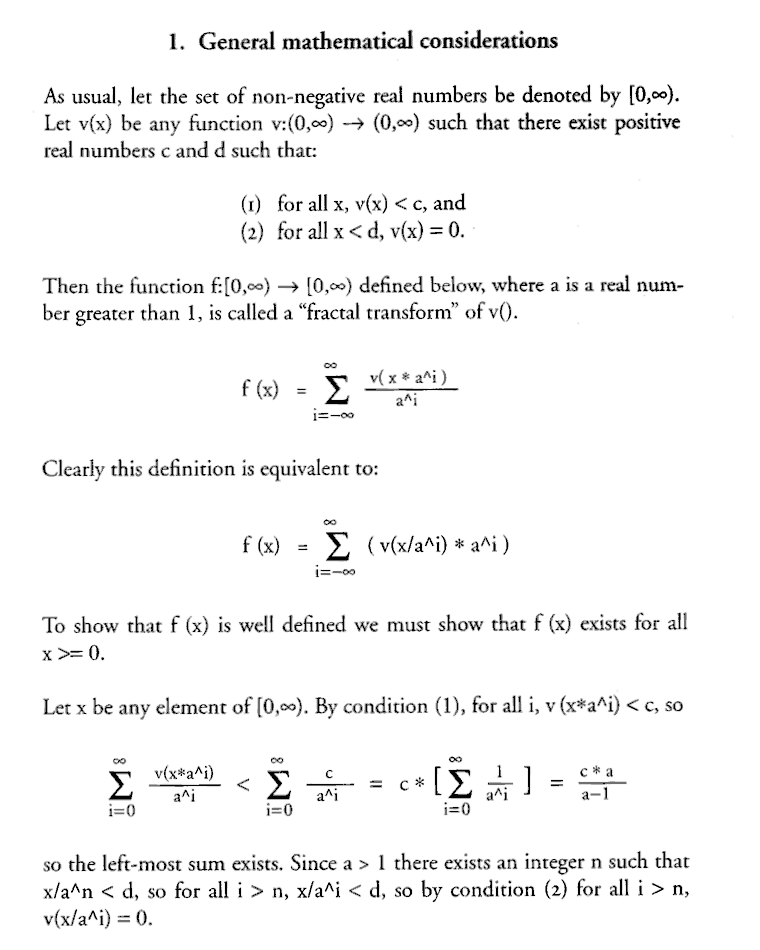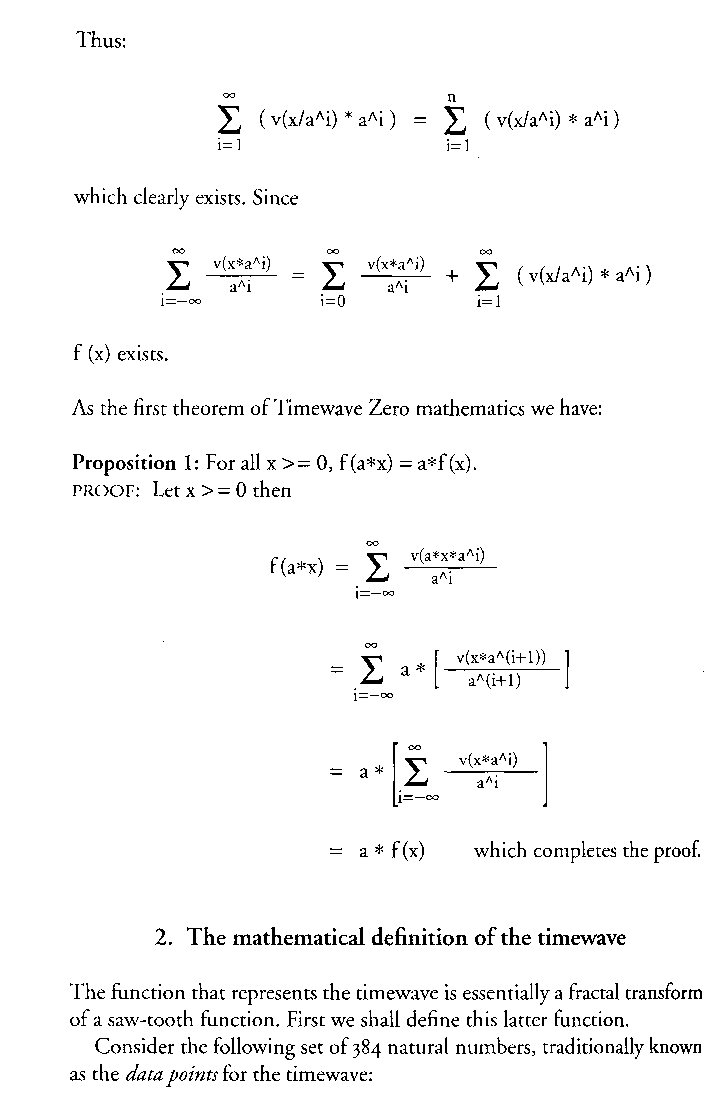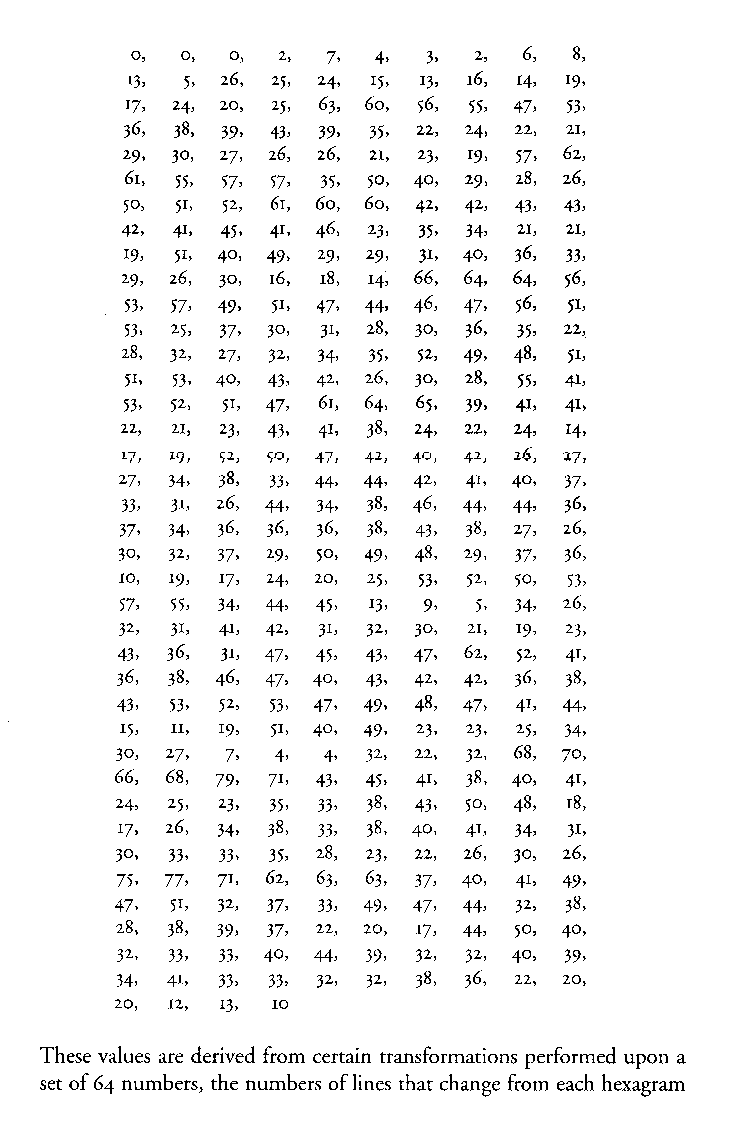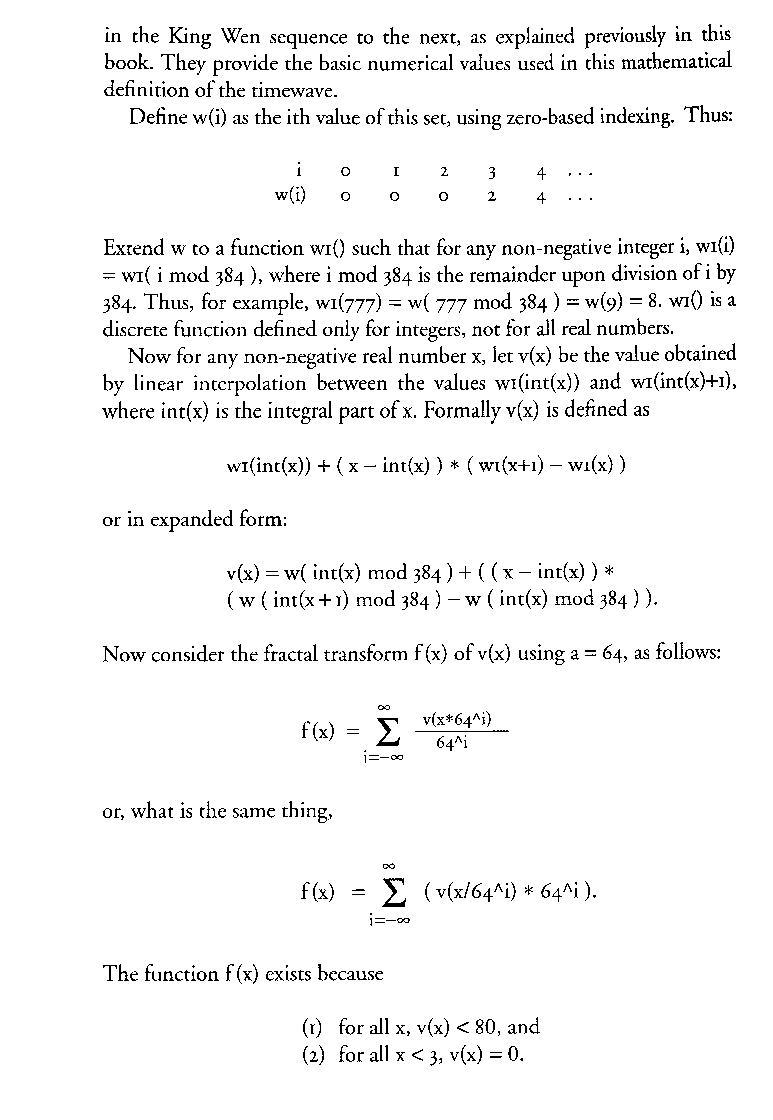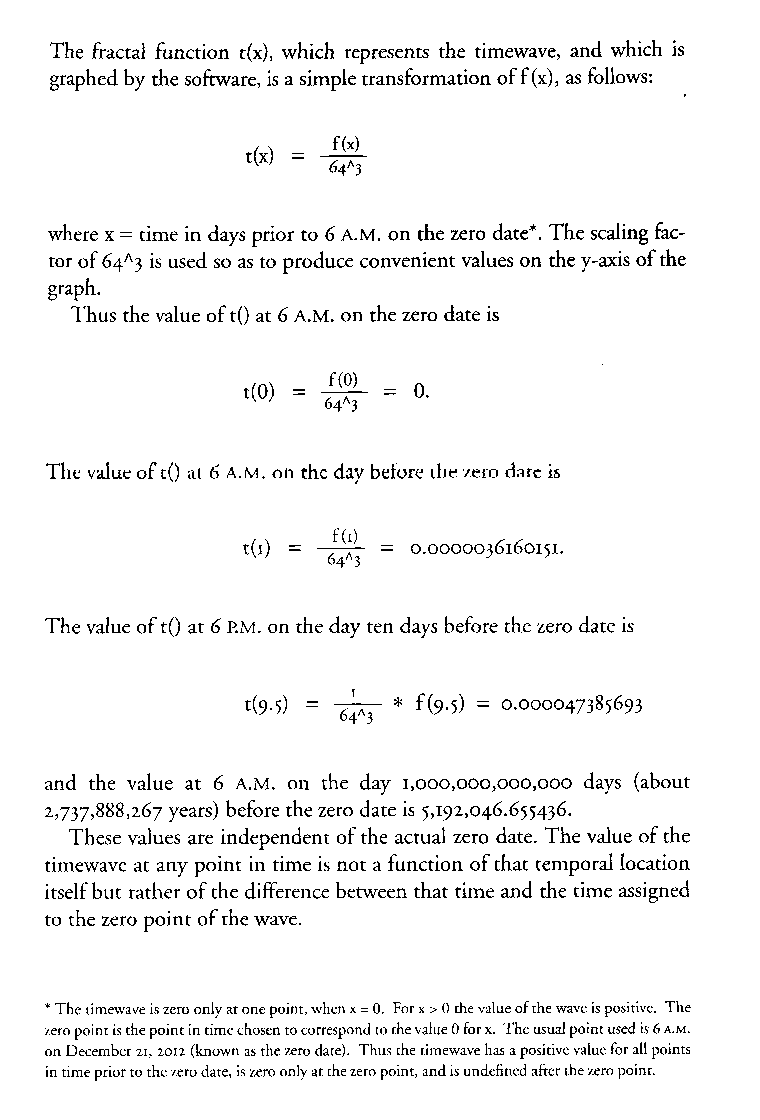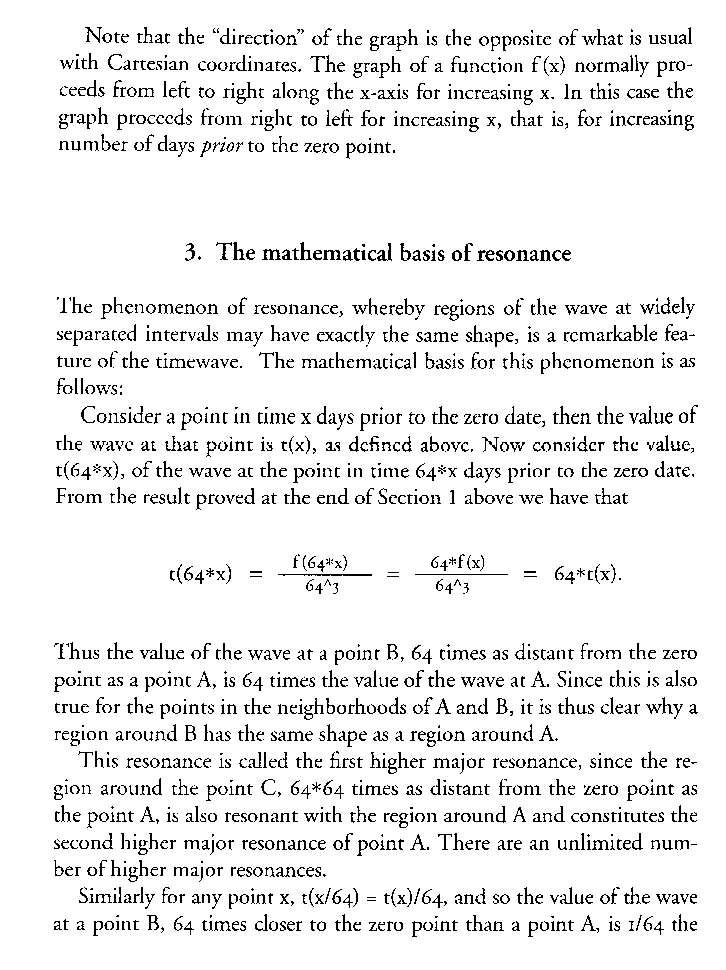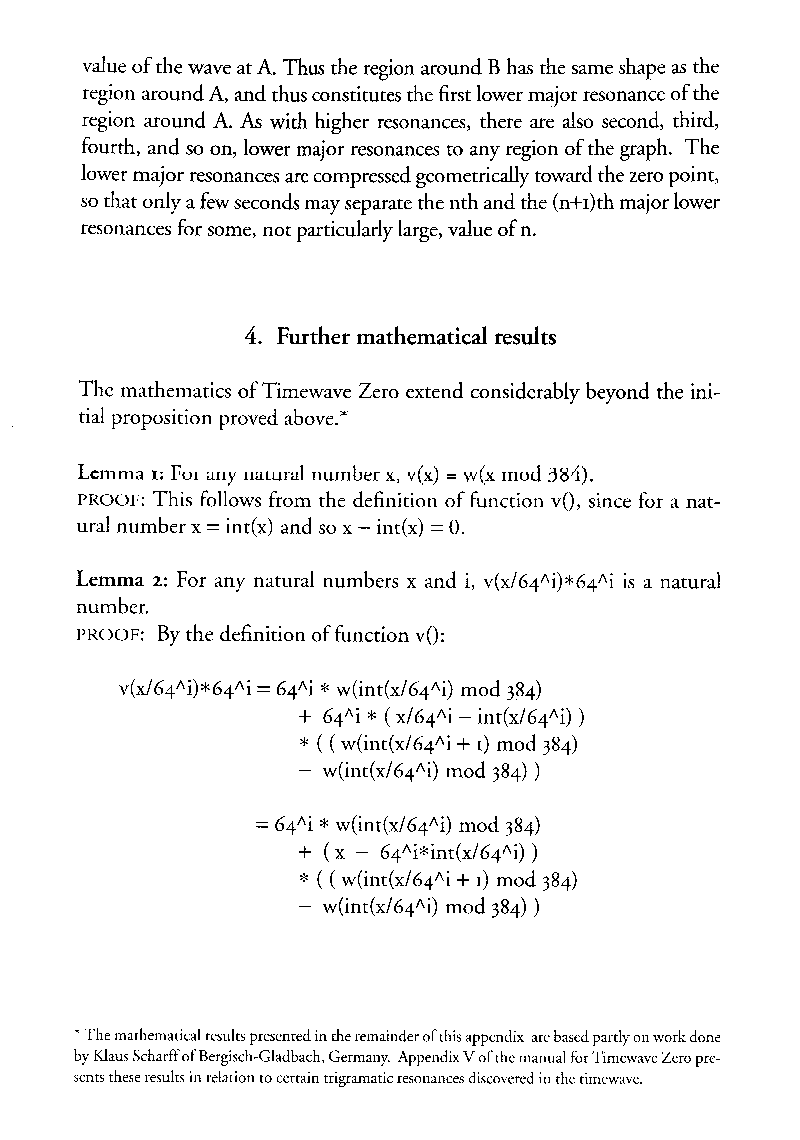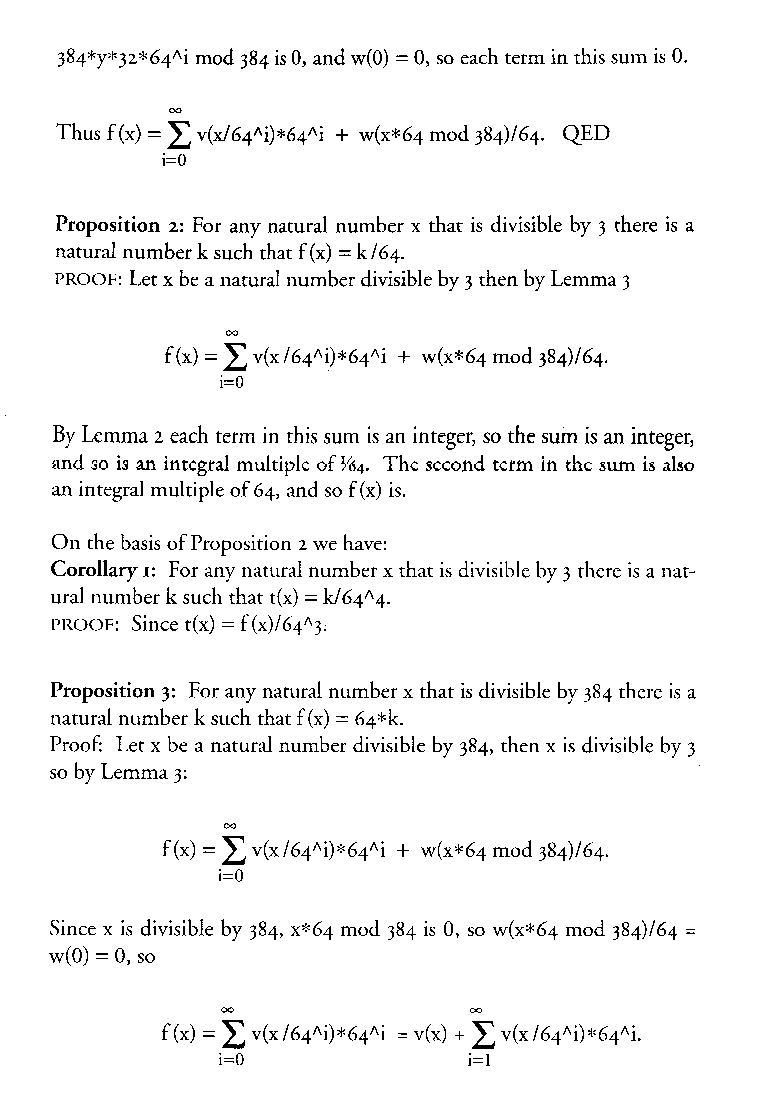The Mathematics of Timewave Zero

This article, written by Peter Meyer, first appeared as an appendix (pages 211-220) in The Invisible Landscape (2nd edition, HarperCollins, 1993). Although there are objections to the timewave zero theory considered as a scientific theory, the mathematics underlying the theory is valid. The theory may be explored in detail by use of the Fractal Time software.

It should be noted that the construction of the fractal timewave starting from the King Wen Sequence of I Ching hexagrams is a 2-step process. The first, described in Derivation of the Timewave from the King Wen Sequence of Hexagrams, consists in the construction of a set of 384 numbers. The second, described in this article, consists in the construction of a fractal function (which, when graphed, is the timewave) based on any set of 384 numbers satisfying certain properties, a set which might or might not be the numbers generated in the first step (thus allowing alternative timewaves),